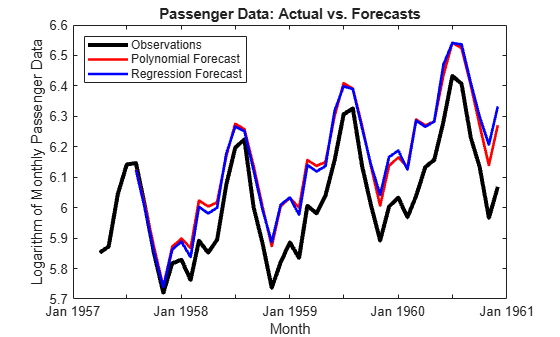# Model Seasonal Lag Effects Using Indicator Variables

This example shows how to estimate a seasonal ARIMA model:

• Model the seasonal effects using a multiplicative seasonal model.

• Use indicator variables as a regression component for the seasonal effects, called seasonal dummies.

Subsequently, their forecasts show that the methods produce similar results. The time series is monthly international airline passenger numbers from 1949 to 1960.

### Step 1. Load the data.

Load the data set `Data_Airline`, and plot the natural log of the monthly passenger totals counts.

```load('Data_Airline.mat') dat = log(Data); % Transform to logarithmic scale T = size(dat,1); y = dat(1:103); % Estimation sample```

`y` is the part of `dat` used for estimation, and the rest of `dat` is the holdout sample to compare the two models' forecasts.

### Step 2. Define and fit the model specifying seasonal lags.

Create an ARIMA$\left(0,1,1\right)\left(0,1,1{\right)}_{12}$ model

`$\left(1-L\right)\left(1-{L}^{12}\right){y}_{t}=\left(1+{\theta }_{1}L\right)\left(1+{\Theta }_{12}{L}^{12}\right){\epsilon }_{t},$`

where ${\epsilon }_{t}$ is an independent and identically distributed normally distributed series with mean 0 and variance ${\sigma }^{2}$. Use `estimate` to fit `Mdl1` to `y`.

```Mdl1 = arima('Constant',0,'MALags',1,'D',1,... 'SMALags',12,'Seasonality',12); EstMdl1 = estimate(Mdl1,y);```
``` ARIMA(0,1,1) Model Seasonally Integrated with Seasonal MA(12) (Gaussian Distribution): Value StandardError TStatistic PValue ________ _____________ __________ __________ Constant 0 0 NaN NaN MA{1} -0.35732 0.088031 -4.059 4.9286e-05 SMA{12} -0.61469 0.096249 -6.3864 1.6985e-10 Variance 0.001305 0.0001527 8.5467 1.2671e-17 ```

The fitted model is

`$\left(1-L\right)\left(1-{L}^{12}\right){y}_{t}=\left(1-0.357L\right)\left(1-0.615{L}^{12}\right){\epsilon }_{t},$`

where ${\epsilon }_{t}$ is an iid normally distributed series with mean 0 and variance 0.0013.

### Step 3. Define and fit the model using seasonal dummies.

Create an ARIMAX(0,1,1) model with period 12 seasonal differencing and a regression component,

`$\left(1-L\right)\left(1-{L}^{12}\right){y}_{t}=\left(1-0.357L\right)\left(1-0.615{L}^{12}\right){\epsilon }_{t},$`

$\left\{{x}_{t};t=1,...,T\right\}$ is a series of T column vectors having length 12 that indicate in which month observation $t$ was measured. A 1 in row i of ${x}_{t}$ indicates that the observation was measured in month i, the rest of the elements are 0s.

Note that if you include an additive constant in the model, then the T rows of the design matrix `X` are composed of the row vectors $\left[\begin{array}{cc}1& {x}_{t}^{\prime }\end{array}\right]$. Therefore, `X` is rank deficient, and one regression coefficient is not identifiable. A constant is left out of this example to avoid distraction from the main purpose. Format the in-sample X matrix

```X = dummyvar(repmat((1:12)',12,1)); % Format the presample X matrix X0 = [zeros(1,11) 1; dummyvar((1:12)')]; Mdl2 = arima('Constant',0,'MALags',1,'D',1,... 'Seasonality',12); EstMdl2 = estimate(Mdl2,y,'X',[X0; X]);```
``` ARIMAX(0,1,1) Model Seasonally Integrated (Gaussian Distribution): Value StandardError TStatistic PValue __________ _____________ __________ __________ Constant 0 0 NaN NaN MA{1} -0.40711 0.084387 -4.8242 1.4053e-06 Beta(1) -0.002577 0.025168 -0.10239 0.91845 Beta(2) -0.0057769 0.031885 -0.18118 0.85623 Beta(3) -0.0022034 0.030527 -0.072179 0.94246 Beta(4) 0.00094737 0.019867 0.047687 0.96197 Beta(5) -0.0012146 0.017981 -0.067551 0.94614 Beta(6) 0.00487 0.018374 0.26505 0.79097 Beta(7) -0.0087944 0.015285 -0.57535 0.56505 Beta(8) 0.0048346 0.012484 0.38728 0.69855 Beta(9) 0.001437 0.018245 0.078758 0.93722 Beta(10) 0.009274 0.014751 0.62869 0.52955 Beta(11) 0.0073665 0.0105 0.70158 0.48294 Beta(12) 0.00098841 0.014295 0.069146 0.94487 Variance 0.0017715 0.00024657 7.1848 6.7329e-13 ```

The fitted model is

`$\left(1-L\right)\left(1-{L}^{12}\right){y}_{t}={X}_{t}\underset{}{\overset{ˆ}{\beta }}+\left(1-0.407L\right){\epsilon }_{t},$`

where ${\epsilon }_{t}$ is an iid normally distributed series with mean 0 and variance 0.0017 and $\underset{}{\overset{ˆ}{\beta }}$ is a column vector with the values `Beta1` - `Beta12`. Note that the estimates `MA{1}` and `Variance` between `Mdl1` and `Mdl2` are not equal.

### Step 4. Forecast using both models.

Use `forecast` to forecast both models 41 periods into the future from July 1957. Plot the holdout sample using these forecasts.

```yF1 = forecast(EstMdl1,41,y); yF2 = forecast(EstMdl2,41,y,'X0',X(1:103,:),'XF',X(104:end,:)); l1 = plot(100:T,dat(100:end),'k','LineWidth',3); hold on l2 = plot(104:144,yF1,'-r','LineWidth',2); l3 = plot(104:144,yF2,'-b','LineWidth',2); hold off title('Passenger Data: Actual vs. Forecasts') xlabel('Month') ylabel('Logarithm of Monthly Passenger Data') legend({'Observations','Polynomial Forecast',... 'Regression Forecast'},'Location','NorthWest')```Though they overpredict the holdout observations, the forecasts of both models are almost equivalent. One main difference between the models is that `EstMdl1` is more parsimonious than `EstMdl2`.

References:

Box, G. E. P., G. M. Jenkins, and G. C. Reinsel. Time Series Analysis: Forecasting and Control. 3rd ed. Englewood Cliffs, NJ: Prentice Hall, 1994.